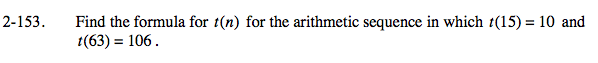### Home > AC > Chapter 13 > Lesson 13.RE3-S > Problem2-153

2-153.Visualize the sequences as two points on a graph, (15, 10) and (63, 106).

Finding the common difference is the same as finding the constant rate of change of a line, the slope.

Use the slope or common difference and one of the points, say (15, 10) to substitute into t(n) = mn + b. And solve: 10 = 2(15) + b

t(n) = 2n − 20